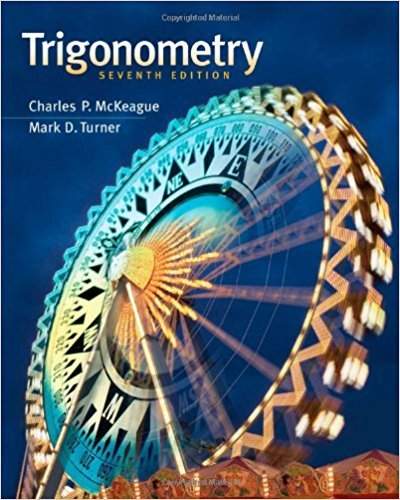×

×

# Solutions for Chapter 6.2: More on Trigonometric Equations## Full solutions for Trigonometry | 7th Edition

ISBN: 9781111826857Solutions for Chapter 6.2: More on Trigonometric Equations

Solutions for Chapter 6.2
4 5 0 405 Reviews
23
1
##### ISBN: 9781111826857

Chapter 6.2: More on Trigonometric Equations includes 83 full step-by-step solutions. Since 83 problems in chapter 6.2: More on Trigonometric Equations have been answered, more than 27999 students have viewed full step-by-step solutions from this chapter. This expansive textbook survival guide covers the following chapters and their solutions. This textbook survival guide was created for the textbook: Trigonometry, edition: 7. Trigonometry was written by and is associated to the ISBN: 9781111826857.

Key Math Terms and definitions covered in this textbook
• Change of basis matrix M.

The old basis vectors v j are combinations L mij Wi of the new basis vectors. The coordinates of CI VI + ... + cnvn = dl wI + ... + dn Wn are related by d = M c. (For n = 2 set VI = mll WI +m21 W2, V2 = m12WI +m22w2.)

• Characteristic equation det(A - AI) = O.

The n roots are the eigenvalues of A.

• Determinant IAI = det(A).

Defined by det I = 1, sign reversal for row exchange, and linearity in each row. Then IAI = 0 when A is singular. Also IABI = IAIIBI and

• Eigenvalue A and eigenvector x.

Ax = AX with x#-O so det(A - AI) = o.

• Free variable Xi.

Column i has no pivot in elimination. We can give the n - r free variables any values, then Ax = b determines the r pivot variables (if solvable!).

• Full row rank r = m.

Independent rows, at least one solution to Ax = b, column space is all of Rm. Full rank means full column rank or full row rank.

• Hermitian matrix A H = AT = A.

Complex analog a j i = aU of a symmetric matrix.

• Normal equation AT Ax = ATb.

Gives the least squares solution to Ax = b if A has full rank n (independent columns). The equation says that (columns of A)·(b - Ax) = o.

• Nullspace matrix N.

The columns of N are the n - r special solutions to As = O.

• Plane (or hyperplane) in Rn.

Vectors x with aT x = O. Plane is perpendicular to a =1= O.

• Positive definite matrix A.

Symmetric matrix with positive eigenvalues and positive pivots. Definition: x T Ax > 0 unless x = O. Then A = LDLT with diag(D» O.

• Projection matrix P onto subspace S.

Projection p = P b is the closest point to b in S, error e = b - Pb is perpendicularto S. p 2 = P = pT, eigenvalues are 1 or 0, eigenvectors are in S or S...L. If columns of A = basis for S then P = A (AT A) -1 AT.

• Projection p = a(aTblaTa) onto the line through a.

P = aaT laTa has rank l.

• Pseudoinverse A+ (Moore-Penrose inverse).

The n by m matrix that "inverts" A from column space back to row space, with N(A+) = N(AT). A+ A and AA+ are the projection matrices onto the row space and column space. Rank(A +) = rank(A).

• Schur complement S, D - C A -} B.

Appears in block elimination on [~ g ].

• Simplex method for linear programming.

The minimum cost vector x * is found by moving from comer to lower cost comer along the edges of the feasible set (where the constraints Ax = b and x > 0 are satisfied). Minimum cost at a comer!

• Solvable system Ax = b.

The right side b is in the column space of A.

• Spanning set.

Combinations of VI, ... ,Vm fill the space. The columns of A span C (A)!

• Special solutions to As = O.

One free variable is Si = 1, other free variables = o.

• Volume of box.

The rows (or the columns) of A generate a box with volume I det(A) I.